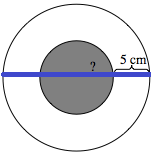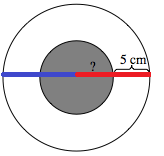### Home > MC2 > Chapter 6 > Lesson 6.1.1 > Problem6-11

6-11.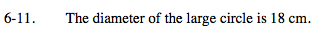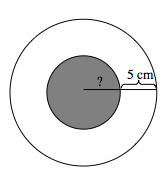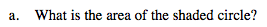Identify what part of the diagram 18 cm represents.

What would the radius of the large circle be?

Notice that the radius of the larger circle is 5 cm more than the radius of the shaded circle.

Subtract the radius of the large circle by 5 cm to find the radius of the shaded circle: 9 cm − 5 cm = 4 cm. Now that you have the radius of the shaded circle, recall how to use that to find the area.

A = r2π

A = (4)2π
The area is approximately 50.27 cm2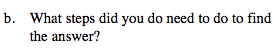Explain what you did in part (a).